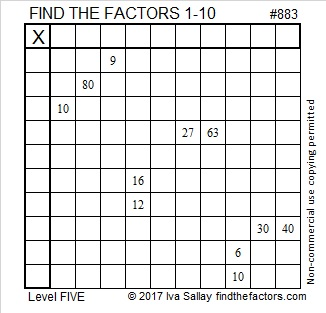# 883 and Level 5

833 is the sum of the eleven prime numbers from 59 to 103.

833 is also the sum of these three consecutive prime numbers: 283 + 293 + 307 = 833.

883 is palindrome 373 in BASE 16 because 3(16²) + 7(16) + 3(1) = 883.Print the puzzles or type the solution on this excel file: 10-factors-875-885

• 883 is a prime number.
• Prime factorization: 883 is prime.
• The exponent of prime number 883 is 1. Adding 1 to that exponent we get (1 + 1) = 2. Therefore 883 has exactly 2 factors.
• Factors of 883: 1, 883
• Factor pairs: 883 = 1 × 883
• 883 has no square factors that allow its square root to be simplified. √883 ≈ 29.7153159How do we know that 883 is a prime number? If 883 were not a prime number, then it would be divisible by at least one prime number less than or equal to √883 ≈ 29.7. Since 883 cannot be divided evenly by 2, 3, 5, 7, 11, 13, 17, 19, 23, or 29, we know that 883 is a prime number.

This site uses Akismet to reduce spam. Learn how your comment data is processed.